# Summing Amplifier or Op Amp Adder

An op amp is an amplifier. But an op amp can also perform summing operation. We can design an op amp circuit to combine number of input signals and to produce single output as a weighted sum of input signals.

Summing amplifier is basically an op amp circuit that can combine numbers of input signal to a single output that is the weighted sum of the applied inputs.

The summing Amplifier is one variation of inverting amplifier. In inverting amplifier there is only one voltage signal applied to the inverting input as shown below,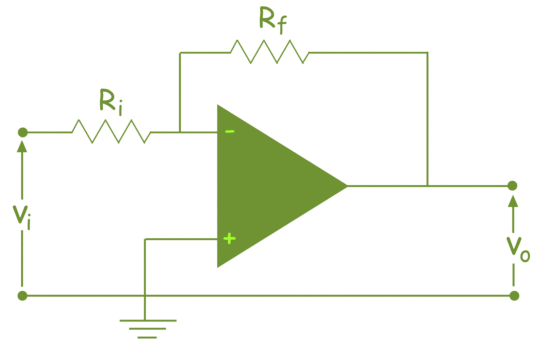This simple inverting amplifier can easily be modified to summing amplifier, if we connect several input terminals in parallel to the existing input terminals as shown below.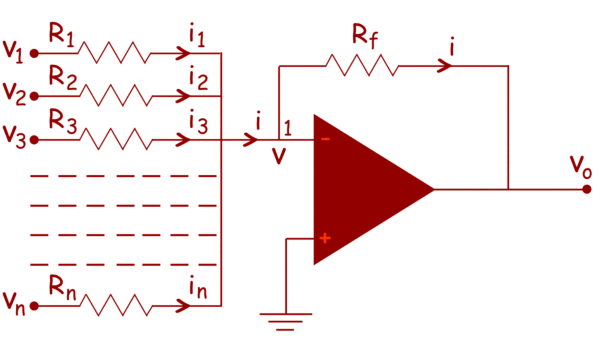Here, n numbers of input terminal are connected in parallel. Here, in the circuit, the non-inverting terminal of the op amp is grounded, hence potential at that terminal is zero. As the op amp is considered as ideal op amp, the potential of the inverting terminal is also zero.
So, the electric potential at node 1, is also zero. From the circuit, it is also clear that the current i is the sum of currents of input terminals.

Therefore,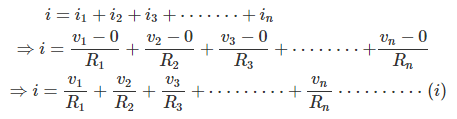Now, in the case of ideal op amp the current at the inverting and non-inverting terminal are zero. So, as per Kirchhoff Current Law, the entire input current passes through the feedback path of resistance Rf. That means,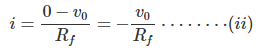From, equation (i) and (ii), we get,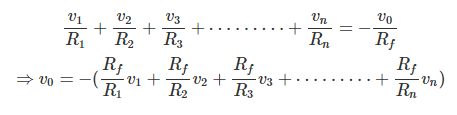This indicates that output voltage v0 is weighted sum of numbers of input voltages.

## Example of summing Amplifier

Let us calculate the output voltage of 3 inputs summer or summing amplifier, circuit as shown below,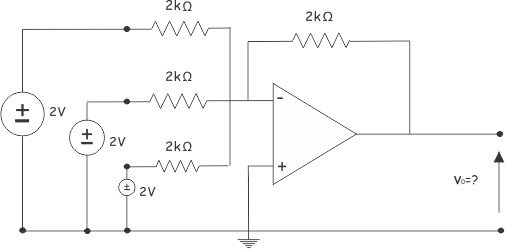Here, as per equation of summing amplifier,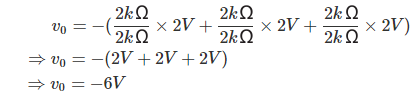Want To Learn Faster? 🎓
Get electrical articles delivered to your inbox every week.
No credit card required—it’s 100% free.